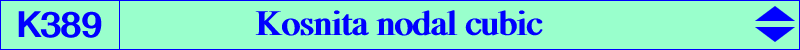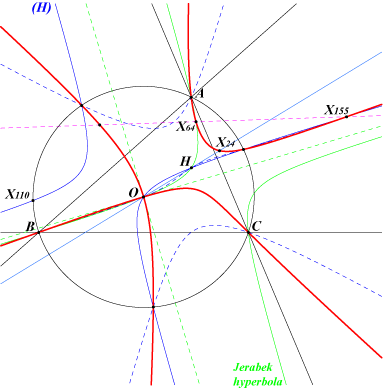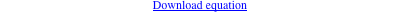too complicated to be written here. Click on the link to download a text file.X(3), X(24), X(64), X(155), X(2353), X(3053), X(3435), X(14264), X(32542), X(37579), X(51895), X(52041), X(52168) points of the Orthocubic on the circumcircle infinite points of pK(X6, X12111) vertices of the tangential triangleK389 is the third polar of O in the Kosnita quartic Q064. It is also the Phi-transform of the rectangular hyperbola (H) with center X(113) passing through X(3), X(4), X(110), X(155), X(1351), X(1352), X(2574), X(2575) which is the reflection of the Jerabek hyperbola in the nine point center X(5). See CL037. Hence it is possible to map the Euler line onto K389 : for M on the Euler line, M* lies on the Jerabek hyperbola, its reflection N' in X(5) lies on (H) and Phi(N') = N lies on K389. This same composition of three involutions naturally maps K389 back to the Euler line. This gives hundreds of centers on the cubic. The isogonal transform of K389 is K617. K389 is actually the generalized Lemoine cubic with node O of the tangential triangle. The nodal tangents are parallel at O to the asymptotes of the Jerabek hyperbola. See also another characterization and a generalization at Q098.